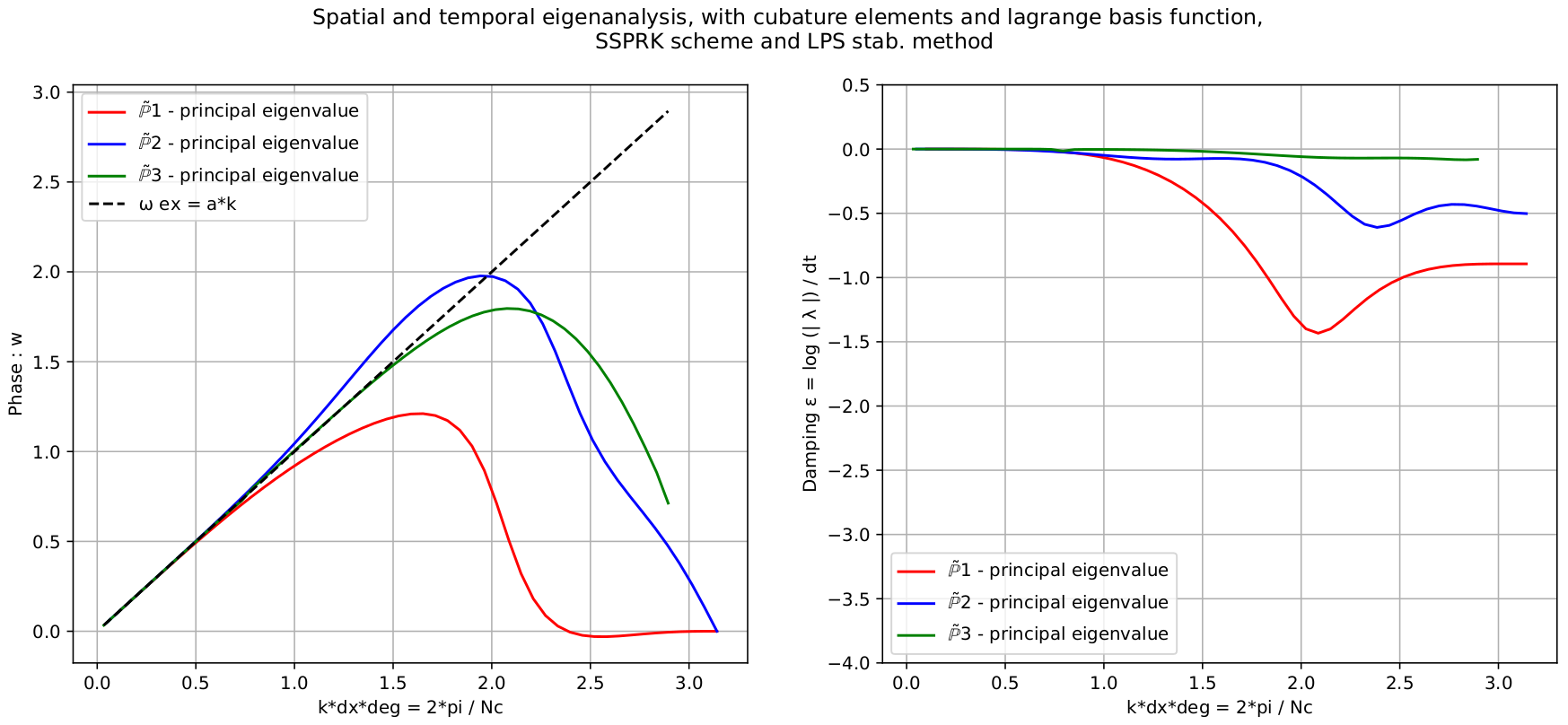# Spectral analysis of continuous FEM for hyperbolic PDEs: influence of approximation, stabilization, and time-stepping

Published in arXiv, 2021

Recommended citation: Michel, S., Torlo, D., Ricchiuto, M. and Abgrall, R.. Spectral Analysis of Continuous FEM for Hyperbolic PDEs: Influence of Approximation, Stabilization, and Time-Stepping. J Sci Comput 89, 31 (2021). https://doi.org/10.1007/s10915-021-01632-7 https://doi.org/10.1007/s10915-021-01632-7

We study continuous finite element dicretizations for one dimensional hyperbolic partial differential equations. The main contribution of the paper is to provide a fully discrete spectral analysis, which is used to suggest optimal values of the CFL number and of the stabilization parameters involved in different types of stabilization operators. In particular, we analyze the streamline-upwind Petrov-Galerkin (SUPG) stabilization technique, the continuous interior penalty (CIP) stabilization method and the local projection stabilization (LPS). Three different choices for the continuous finite element space are compared: Bernstein polynomials, Lagrangian polynomials on equispaced nodes, and Lagrangian polynomials on Gauss-Lobatto cubature nodes. For the last choice, we only consider inexact quadrature based on the formulas corresponding to the degrees of freedom of the element, which allows to obtain a fully diagonal mass matrix. We also compare different time stepping strategies, namely Runge-Kutta (RK), strong stability preserving RK (SSPRK) and deferred correction time integration methods. The latter allows to alleviate the computational cost as the mass matrix inversion is replaced by the high order correction iterations. To understand the effects of these choices, both time-continuous and fully discrete Fourier analysis are performed. These allow to compare all the different combinations in terms of accuracy and stability, as well as to provide suggestions for optimal values discretization parameters involved. The results are thoroughly verified numerically both on linear and non-linear problems, and error-CPU time curves are provided. Our final conclusions suggest that cubature elements combined with SSPRK and CIP or LPS stabilization are the most promising combinations.

DOI https://doi.org/10.1007/s10915-021-01632-7

Arxiv page

Paper PDF